# Exploratory Data Analysis on Iris Dataset

## Introduction

In Machine Learning and Data Science Exploratory Data Analysis is the process of examining a data set and summarizing its main characteristics about it. It may include visual methods to better represent those characteristics or have a general understanding of the dataset. It is a very essential step in a Data Science lifecycle, often consuming a certain time.

In this article, we are going to see some of the characteristics of the Iris dataset through Exploratory Data Analysis.

## The Iris Dataset

The Iris Dataset is very simple often referred to as the Hello World. The dataset has 4 features of three different species of flowers namely Iris setosa, Iris virginica, and Iris versicolor. These features are sepal length, sepal width, petal length, and petal width. There are 150 data points in the dataset, 50 data points for each species.

## EDA on Iris Dataset

First, let's load the dataset from the CSV file "iris_csv.csv" using pandas and have a general overview of it.

https://datahub.io/machine-learning/iris/r/iris.csv

## Code Implementation

### Example 1

import pandas as pd
import numpy as np
import seaborn as sns
import matplotlib.pyplot as plt
%matplotlib inline



sepallength

sepalwidth

petallength

petalwidth

class

0

5.1

3.5

1.4

0.2

Iris-setosa

1

4.9

3.0

1.4

0.2

Iris-setosa

3

4.6

3.1

1.5

0.2

Iris-setosa

4

5.0

3.6

1.4

0.2

Iris-seto

### Example 2

df.info()

RangeIndex: 150 entries, 0 to 149
Data columns (total 5 columns):
#   Column       Non-Null Count  Dtype
---  ------       --------------  -----
0   sepallength  150 non-null    float64
1   sepalwidth   150 non-null    float64
2   petallength  150 non-null    float64
3   petalwidth   150 non-null    float64
4   class        150 non-null    object
dtypes: float64(4), object(1)
memory usage: 6.0+ KB
df.shape

(150, 5)

df.describe()


sepallength

sepalwidth

petallength

petalwidth

count

150.000000

150.000000

150.000000

150.000000

mean

5.843333

3.054000

3.758667

1.198667

std

0.828066

0.433594

1.764420

0.763161

min

4.300000

2.000000

1.000000

0.100000

25%

5.100000

2.800000

1.600000

0.300000

50%

5.800000

3.000000

4.350000

1.300000

max

7.900000

4.400000

6.900000

2.500000

### Example 3

## checking for null values

df.isnull().sum()

sepallength    0
sepalwidth     0
petallength    0
petalwidth     0
class          0
dtype: int64

## Univariate analysis
df.groupby('class').agg(['mean', 'median'])  # passing a list of recognized strings
df.groupby('class').agg([np.mean, np.median])

sepallength sepalwidth petallength petalwidth
mean median mean median mean median mean median
class
Irissetosa 5.006 5.0 3.418 3.4 1.464 1.50 0.244 0.2
Irisversicolor 5.936 5.9 2.770 2.8 4.260 4.35 1.326 1.3
Irisvirginica 6.588 6.5 2.974 3.0 5.552 5.55 2.026 2.0

### Example 4

## Box plot
plt.figure(figsize=(8,4))
sns.boxplot(x='class',y='sepalwidth',data=df ,palette='YlGnBu')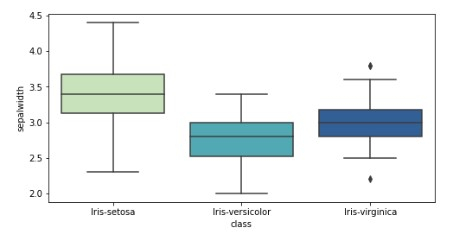### Example 5

## Distribution of particular species
sns.distplot(a=df['petalwidth'], bins=40, color='b')
plt.title('petal width distribution plot')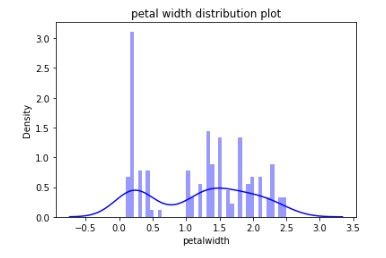### Example 6

## count of number of observation of each species

sns.countplot(x='class',data=df)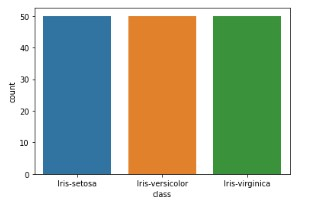### Example 7

## Correlation map using a heatmap matrix

sns.heatmap(df.corr(), linecolor='white', linewidths=1)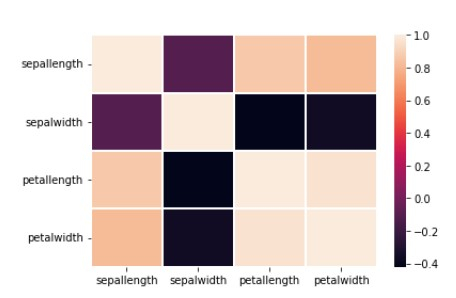### Example 8

## Multivariate analysis – analyis between two or more variable or features
## Scatter plot to see the relation between two or more features like sepal length, petal length,etc
axis = plt.axes()

axis.scatter(df.sepallength, df.sepalwidth)

axis.set(xlabel='Sepal_Length (cm)',
ylabel='Sepal_Width (cm)',
title='Sepal-Length vs Width');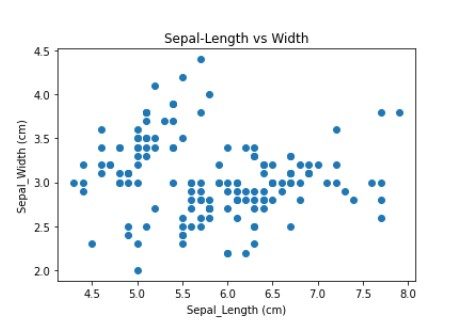### Example 9

sns.scatterplot(x='sepallength', y='sepalwidth', hue='class', data=df,
plt.show()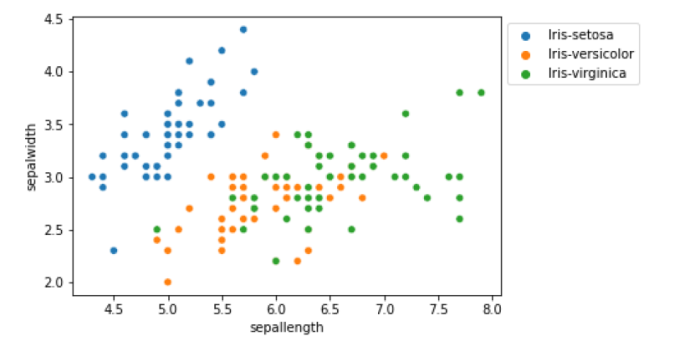### Example 10

## From the above graph we can see that
# Iris-virginica has a longer sepal length while Iris-setosa has larger sepal width
# For setosa sepal width is more than sepal length
## Below is the Frequency histogram plot of all features
axis = df.plot.hist(bins=30, alpha=0.5)
axis.set_xlabel('Size in cm');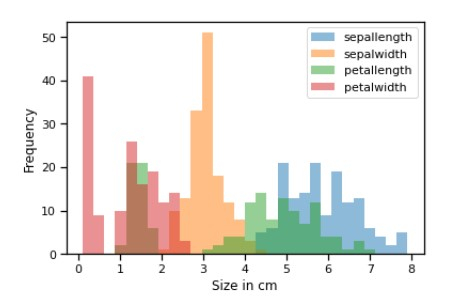### Example 11

# From the above graph we can see that sepalwidth is longer than any other feature followed by petalwidth
## examining correlation
sns.pairplot(df, hue='class')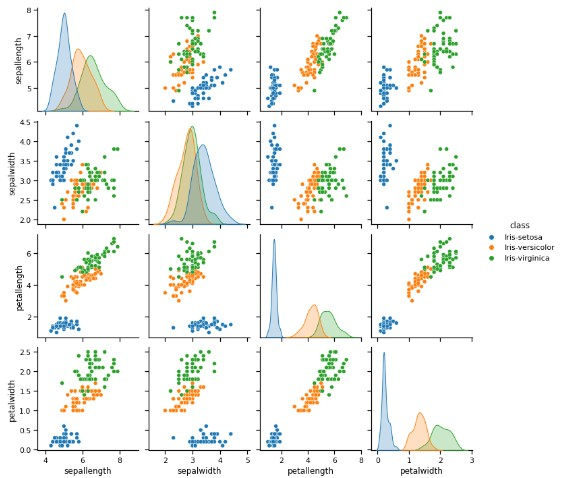### Example 12

figure, ax = plt.subplots(2, 2, figsize=(8,8))

ax[0,0].set_title("sepallength")
ax[0,0].hist(df['sepallength'], bins=8)

ax[0,1].set_title("sepalwidth")
ax[0,1].hist(df['sepalwidth'], bins=6);

ax[1,0].set_title("petallength")
ax[1,0].hist(df['petallength'], bins=5);

ax[1,1].set_title("petalwidth")
ax[1,1].hist(df['petalwidth'], bins=5);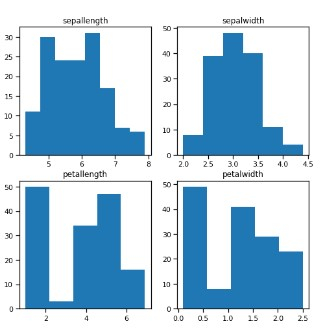### Example 13

# From the above plot we can see that –
# - Sepal length highest freq lies between 5.5 cm to 6 cm which is 30-35 cm
# - Petal length highest freq lies between 1 cm to 2 cm which is 50 cm
# - Sepal width highest freq lies between 3 cm to 3.5 cm which is 70 cm
# - Petal width highest freq lies between 0 cm to 0.5 cm which is 40-45 cm


## Conclusion

Exploratory Data Analysis is extremely used by both Data Scientists and Analysts. It tells a lot about the characteristics of the given data, its distribution, and how it can be useful.